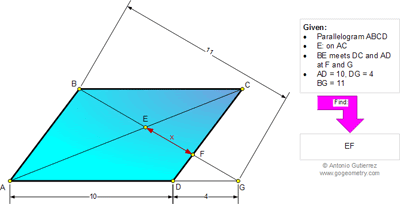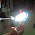## Tuesday, February 11, 2014

### Geometry Problem 978: Parallelogram, Diagonal, Transversal, Similarity, Metric Relations

Geometry Problem
Level: Mathematics Education, High School, Honors Geometry, College.

Click the figure below to see the complete problem 978.#### 2 comments:

1.GF/11=4/14 because DFG is similar to ABG. Also BE/(11-BE)=10/(10+4) because EBC is similar to EGA which makes GF=22/7 and BE=55/12, so x=11-55/12-22/7=275/84

2.We use Barycentric Coordinates
Definition: Capital letters will denote vectors. P = x A + y B + z C will be written as P = (x, y, z). By definition, x + y + z = 1
Let A = (1, 0, 0), B = (0, 1, 0) and C = (0, 0, 1).
A + C = B + D, because ABCD is a parallelogram. This implies that D = (1, -1, 1).
AD : DG = 10 : 4, and with division of an interval in vector form, it’s obvious that 2A/7 + 5G/7 = D.
With a few rearrangements, G = (1, -7/5, 7/5).
If (x, y, z) is on a specified straight line, then 0 = ux + vy + wz for some constant u, v, w. After solving the systems of equations, it’s clear that line CD is x + y = 0, line BG is 7x/5 = z, line AC is y = 0.
Intersection of CD and BG is F. Using the fact that x + y + z = 1, it’s evident that F = (5/7, -5/7, 1).
Similarly, E = (5/12, 7/12).
With tedious algebra, it’s true that:
5B/12 + 7F/12 = E
25G/49 + 24E/49 = F
We can deduce from this that BE : FE = 7 : 5, FG : FE = 24 : 25
Hence, BE : FE : FG = 35 : 25 : 24
So FE : BG = 25 : 84, and given that BG = 11, it’s clear that EF = 275/84.